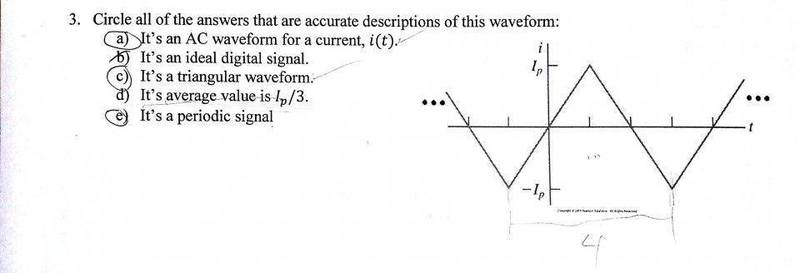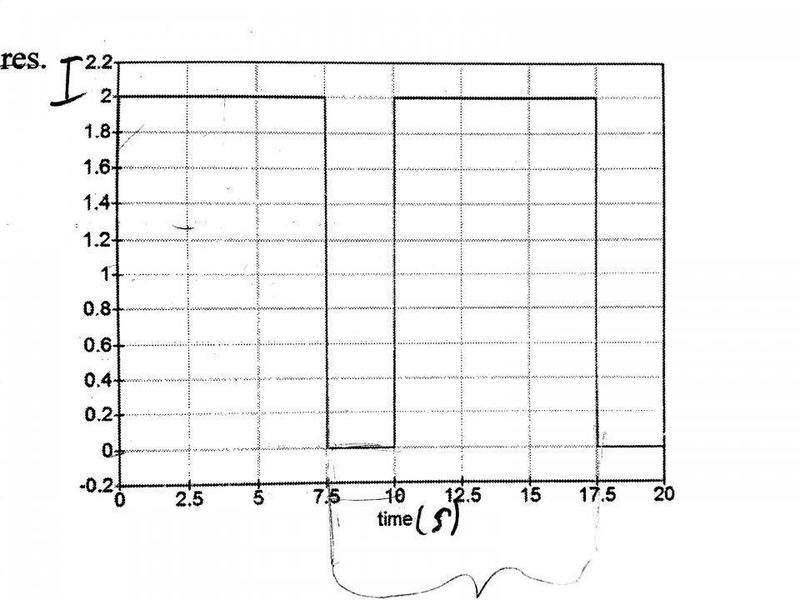Waveform and Average Values

Homework Statement

Circle all that are correct

V(t)=Vocos(wt)

The Attempt at a Solution

I don't really understand what the question wants when they ask for an average value. Average value of what? And how do you find it. There are a lot of questions with graphs like this that ask to find the average value, but I'm not sure how to get that exactly. How do I find the average value of this problem?NascentOxygen
Staff Emeritus
On a graph, the average value is drawn as a horizontal straight line at a level such that there is as much enclosed area above that line and the waveform as there is below that line and the waveform, for one cycle.

On a graph, the average value is drawn as a horizontal straight line at a level such that there is as much enclosed area above that line and the waveform as there is below that line and the waveform, for one cycle.
Oh. So do you get the average by just looking at it or is there a formula to get it. Looking at this one it looks like the average would be 0 right? How can I get the average for something like this:NascentOxygen
Staff Emeritus
The easy ones you can do by inspection or by counting squares, but for the harder ones you need to use integral calculus.

Did you plot a horizontal line at y=0 on that graph, to check that it looks about right?

The easy ones you can do by inspection or by counting squares, but for the harder ones you need to use integral calculus.
This is a non-calc based course, so is there an easier way. I've taken up to calc 3 and some diff eq, but I don't think we can use calc for this. (This is EGR 110 - Intro to comp E and EE) Also, the way my teacher does it doesn't make sense... :/

Did you plot a horizontal line at y=0 on that graph, to check that it looks about right?
I looked at one cycle and saw that the triangles would cancel each other out, so it's average would be 0. I think.

NascentOxygen
Staff Emeritus
For the triangular waveform, yes, its average is zero.

For the triangular waveform, yes, its average is zero.
How about for the other one? Is there a simple way of doing it?

NascentOxygen
Staff Emeritus
How about for the other one? Is there a simple way of doing it?
Counting squares. Half will be above the average mark, half below it.

Counting squares. Half will be above the average mark, half below it.
Where would the average mark go? Horizontal line at 1Amp?

NascentOxygen
Staff Emeritus
Where would the average mark go? Horizontal line at 1Amp?
How many "squares" do you count between that line and the waveform?

How many "squares" do you count between that line and the waveform?
Which line? The horizontal line at 1Amp? 7.5 boxes

NascentOxygen
Staff Emeritus